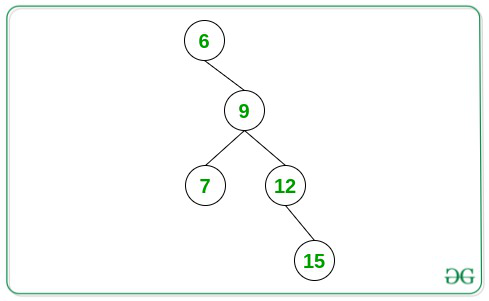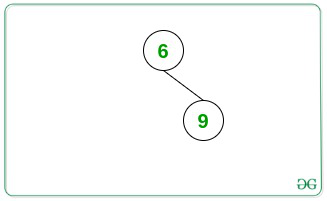Longest path to the bottom of a Binary Tree forming an Arithmetic Progression

• Difficulty Level : Medium
• Last Updated : 20 Aug, 2021

Given a Binary Tree consisting of N nodes, the task is to find the length of the longest path from any node to the bottom of the tree such that all the node values form an Arithmetic Progression.

Examples:

Input:Output: 4
Explanation:
From the above given tree, the longest path with node values forming an AP is {6, 9, 12, 15} (Length = 4).

Input:Output: 2

Approach: The given problem can be solved by using recursion and performing the DFS Traversal on the given tree. The idea is to keep track of the difference between the current root node and the next descendant node and update the length of the longest path accordingly. Follow the steps below to solve the given problem:

• Initialize a variable, say maxLength as 1 that stores the maximum length of the path from any node to the bottom of the tree forming an Arithmetic Progression.
• Define a function, say dfs(root, currentDifference, count, maxLength) that takes the current root node, current difference, count of nodes forming AP, and the resultant maximum length as the parameter and perform the following steps:
• If the root’s left node exists, then perform the following steps:
• Find the difference between the value of the root and its left node.
• If the difference is found to be currentDifference, then update the value of maxLength to the maximum of maxLength and (count + 1) and recursively call for the function dfs(root->left, currentDifference, count + 1, maxLength).
• Otherwise, recursively call for the dfs(root->left, difference, 2, maxLength).
• If the root’s right node exists, then perform the following steps:
• Find the difference between the value of the root and its right node.
• If the difference is found to be currentDifference, then update the value of maxLength to the maximum of maxLength and (count + 1) and recursively call for the function dfs(root->right, currentDifference, count + 1, maxLength).
• Otherwise, recursively call for the dfs(root->left, difference, 2, maxLength).
• If the left child of the given root node exists, then call for the dfs(root->left, difference, 2, maxLength) where the difference is the difference between the value of the root and its left node.
• If the right child of the given root node exists, then call for the dfs(root->right, difference, 2, maxLength) where the difference is the difference between the value of the root and its right node.
• After completing the above steps, print the value of maxLength as the resultant maximum length of the path from any node to the bottom of the tree forming an Arithmetic Progression.

Below is the implementation of the above approach:

C++

 // C++ program for the above approach #include "bits/stdc++.h"using namespace std; // Structure of the Tree Nodestruct Tree {    int val;    Tree *left, *right;}; // Function to create a new nodeTree* newNode(int data){    Tree* temp = new Tree();    temp->val = data;    temp->left = temp->right = NULL;    return temp;} // Function to perform DFS Traversal// to find the maximum length of a path// to a bottom node forming an APvoid dfs(Tree* root, int currentDifference,         int count, int& maxLength){    // If the root's left child exists    if (root->left) {         // Calculate the difference        int difference = root->left->val                         - root->val;         // If the difference is same        // as the current difference        if (difference == currentDifference) {            dfs(root->left, currentDifference,                count + 1, maxLength);             // Update the maxLength            maxLength = max(maxLength,                            count + 1);        }         // Otherwise        else {            dfs(root->left, difference,                2, maxLength);        }    }     // If the root's right child exists    if (root->right) {         // Find the difference        int difference = root->right->val                         - root->val;         // If the difference is the same        // as the current difference        if (difference == currentDifference) {             dfs(root->right, currentDifference,                count + 1, maxLength);             // Update the maxLength            maxLength = max(maxLength,                            count + 1);        }         // Otherwise        else {            dfs(root->right, difference,                2, maxLength);        }    }} // Function to find the maximum length// of the path from any node to bottom// of the tree forming an APint maximumLengthAP(Tree* root){     // Base Cases    if (root == NULL)        return 0;     if (root->left == NULL        and root->right == NULL) {        return 1;    }     // Stores the resultant    // maximum length of the path    int maxLength = 2;     // If the root's left child exists    if (root->left) {         int difference = root->left->val                         - root->val;        dfs(root->left, difference, 2,            maxLength);    }     // If the root's right child exists    if (root->right) {        int difference = root->right->val                         - root->val;        dfs(root->right, difference, 2,            maxLength);    }     // Return the maximum length obtained    return maxLength;} // Driver Codeint main(){     // Given Tree    Tree* root = newNode(6);    root->right = newNode(9);    root->right->left = newNode(7);    root->right->right = newNode(12);    root->right->right->right = newNode(15);     cout << maximumLengthAP(root);     return 0;}

Java

 // Java program for the above approachimport java.lang.*;import java.util.*; class GFG{     static int maxLength;        // TreeNode classstatic class Node{    public int val;    public Node left, right;}; static Node newNode(int key){    Node temp = new Node();    temp.val = key;    temp.left = temp.right = null;    return temp;} // Function to perform DFS Traversal// to find the maximum length of a path// to a bottom node forming an APstatic void dfs(Node root, int currentDifference,                int count){         // If the root's left child exists    if (root.left != null)    {                 // Calculate the difference        int difference = root.left.val - root.val;         // If the difference is same        // as the current difference        if (difference == currentDifference)        {            dfs(root.left, currentDifference,                count + 1);             // Update the maxLength            maxLength = Math.max(maxLength,                                 count + 1);        }         // Otherwise        else        {            dfs(root.left, difference, 2);        }    }     // If the root's right child exists    if (root.right != null)    {                 // Find the difference        int difference = root.right.val - root.val;         // If the difference is the same        // as the current difference        if (difference == currentDifference)        {            dfs(root.right, currentDifference,                count + 1);             // Update the maxLength            maxLength = Math.max(maxLength,                                 count + 1);        }         // Otherwise        else        {            dfs(root.right, difference, 2);        }    }} // Function to find the maximum length// of the path from any node to bottom// of the tree forming an APstatic int maximumLengthAP(Node root){         // Base Cases    if (root == null)        return 0;     if (root.left == null &&        root.right == null)    {        return 1;    }     // Stores the resultant    // maximum length of the path     maxLength = 2;     // If the root's left child exists    if (root.left != null)    {        int difference = root.left.val - root.val;        dfs(root.left, difference, 2);    }     // If the root's right child exists    if (root.right != null)    {        int difference = root.right.val - root.val;        dfs(root.right, difference, 2);    }     // Return the maximum length obtained    return maxLength;}    // Driver codepublic static void main(String[] args){     // Given Tree    Node root = newNode(6);    root.right = newNode(9);    root.right.left = newNode(7);    root.right.right = newNode(12);    root.right.right.right = newNode(15);         System.out.println(maximumLengthAP(root));}} // This code is contributed by offbeat

Python3

 # Python3 program for the above approachmaxLength = 2 class Node:       # Constructor to set the data of    # the newly created tree node    def __init__(self, key):        self.val = key        self.left = None        self.right = None # Function to perform DFS Traversal# to find the maximum length of a path# to a bottom node forming an APdef dfs(root, currentDifference, count):         global maxLength     # If the root's left child exists    if (root.left != None):        # Calculate the difference        difference = root.left.val - root.val         # If the difference is same        # as the current difference        if (difference == currentDifference):                     dfs(root.left, currentDifference, count + 1)             # Update the maxLength            maxLength = max(maxLength, count + 1)                  # Otherwise        else:            dfs(root.left, difference, 2)     # If the root's right child exists    if (root.right != None):        # Find the difference        difference = root.right.val - root.val         # If the difference is the same        # as the current difference        if (difference == currentDifference):                     dfs(root.right, currentDifference, count + 1)             # Update the maxLength            maxLength = max(maxLength, count + 1)         # Otherwise        else:            dfs(root.right, difference, 2) # Function to find the maximum length# of the path from any node to bottom# of the tree forming an APdef maximumLengthAP(root):     global maxLength         # Base Cases    if (root == None):        return 0     if (root.left == None and root.right == None):        return 1     # If the root's left child exists    if (root.left != None):        difference = root.left.val - root.val        dfs(root.left, difference, 2)     # If the root's right child exists    if (root.right != None):        difference = root.right.val - root.val        dfs(root.right, difference, 2)     # Return the maximum length obtained    return maxLength # Given Treeroot = Node(6)root.right = Node(9)root.right.left = Node(7)root.right.right = Node(12)root.right.right.right = Node(15)   print(maximumLengthAP(root)) # This code is contributed by decode2207.

C#

 // C# program for the above approachusing System; class GFG{     static int maxLength;        // TreeNode classclass Node{    public int val;    public Node left, right;}; static Node newNode(int key){    Node temp = new Node();    temp.val = key;    temp.left = temp.right = null;    return temp;} // Function to perform DFS Traversal// to find the maximum length of a path// to a bottom node forming an APstatic void dfs(Node root, int currentDifference,                int count){         // If the root's left child exists    if (root.left != null)    {                 // Calculate the difference        int difference = root.left.val - root.val;         // If the difference is same        // as the current difference        if (difference == currentDifference)        {            dfs(root.left, currentDifference,                count + 1);             // Update the maxLength            maxLength = Math.Max(maxLength,                                 count + 1);        }         // Otherwise        else        {            dfs(root.left, difference, 2);        }    }     // If the root's right child exists    if (root.right != null)    {                 // Find the difference        int difference = root.right.val - root.val;         // If the difference is the same        // as the current difference        if (difference == currentDifference)        {            dfs(root.right, currentDifference,                count + 1);             // Update the maxLength            maxLength = Math.Max(maxLength,                                 count + 1);        }         // Otherwise        else        {            dfs(root.right, difference, 2);        }    }} // Function to find the maximum length// of the path from any node to bottom// of the tree forming an APstatic int maximumLengthAP(Node root){         // Base Cases    if (root == null)        return 0;     if (root.left == null &&        root.right == null)    {        return 1;    }     // Stores the resultant    // maximum length of the path     maxLength = 2;     // If the root's left child exists    if (root.left != null)    {        int difference = root.left.val - root.val;        dfs(root.left, difference, 2);    }     // If the root's right child exists    if (root.right != null)    {        int difference = root.right.val - root.val;        dfs(root.right, difference, 2);    }     // Return the maximum length obtained    return maxLength;}    // Driver codepublic static void Main(String[] args){         // Given Tree    Node root = newNode(6);    root.right = newNode(9);    root.right.left = newNode(7);    root.right.right = newNode(12);    root.right.right.right = newNode(15);         Console.WriteLine(maximumLengthAP(root));}} // This code is contributed by gauravrajput1

Javascript


Output:
4

Time Complexity: O(N)
Auxiliary Space: O(1)

My Personal Notes arrow_drop_up